Share

# Balbharati solutions for Textbook for SSC Class 10 Science and Technology part 1 chapter 4 - Effects of electric current [Latest edition]

Textbook page

#### Chapters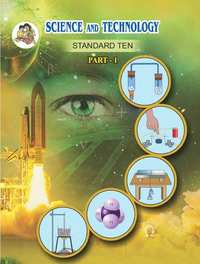## Chapter 4: Effects of electric current

#### Balbharati solutions for Textbook for SSC Class 10 Science and Technology part 1 Chapter 4 Effects of electric current [Pages 60 - 61]

Q 1.1 | Page 60

Tell the odd one out. Give proper explanation.

Fuse wire, bad conductor, rubber gloves, generator.

Q 1.2 | Page 60

Tell the odd one out. Give proper explanation.

Voltmeter, Ammeter, galvanometer, thermometer.

Q 1.3 | Page 60

Tell the odd one out. Give proper explanation.

Loud speaker, microphone, electric motor, magnet.

Q 2.1 | Page 60

Explain the construction and working of the following. Draw a neat diagram and label it.

Electric motor

Q 2.2 | Page 60

Explain the construction and working of the following. Draw a neat diagram and label it.

Electric Generator (AC)

Q 3 | Page 60

Electromagnetic induction means-

a. Charging of an electric conductor.
b. Production of magnetic field due to a current flowing through a coil.
c. Generation of a current in a coil due to relative motion between the coil and the magnet.
d. Motion of the coil around the axle in an electric motor.

Q 4 | Page 60

Explain the difference:
AC generator and DC generator.

Q 5 | Page 60

Which device is used to produce electricity? Describe with a neat diagram.

a. Electric motor
b. Galvanometer
c. Electric Generator (DC)
d. Voltmeter

Q 6 | Page 60

How does the short circuit form? What is its effect?

Q 7.1 | Page 61

Give Scientific reason
Tungsten metal is used to make a solenoid type coil in an electric bulb.

Q 7.2 | Page 61

Give Scientific reason
In the electric equipment producing heat e.g. iron, electric heater, boiler, toaster etc, an alloy such as Nichrome is used, not pure metals.

Q 7.3 | Page 61

Give Scientific reason
For electric power transmission, copper or aluminium wire is used.

Q 7.4 | Page 61

Give Scientific reason
In practice the unit kWh is used for the measurement of electrical energy, rather than joule.

Q 8 | Page 61

Which of the statement given below correctly describes the magnetic field near a long, straight current carrying conductor?

• The magnetic lines of force are in a plane, perpendicular to the conductor in the form of straight lines.

• The magnetic lines of force are parallel to the conductor on all the sides of conductor.

• The magnetic lines of force are perpendicular to the conductor going radially outword.

• The magnetic lines of force are in concentric circles with the wire as the center, in a plane perpendicular to the conductor.

Q 9 | Page 61

What is a solenoid? Compare the magnetic field produced by a solenoid with the magnetic field of a bar magnet. Draw neat figures and name various components.

Q 10.1 | Page 61

Name the following diagram and explain the concept behind them.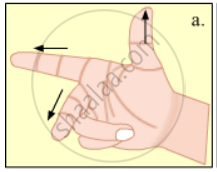Q 10.2 | Page 61

Name the following diagram and explain the concept behind them.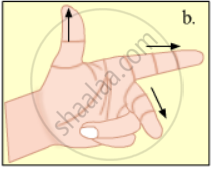Q 11.1 | Page 61

Identify the figures and explain their use.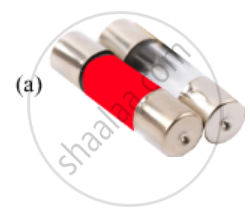Q 11.2 | Page 61

Identify the figures and explain their use.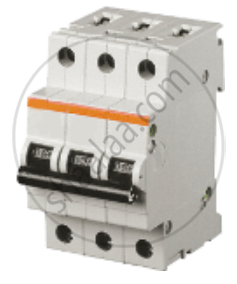Q 11.3 | Page 61

Identify the figures and explain their use.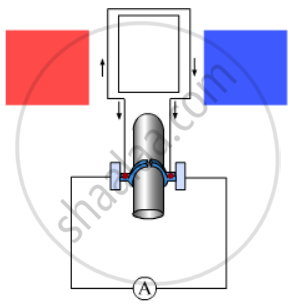Q 12.1 | Page 61

Solve the following example.

Heat energy is being produced in a resistance in a circuit at the rate of 100 W. The current of 3 A is flowing in the circuit. What must be the value of the resistance?

Q 12.2 | Page 61

Solve the following example.

Two tungsten bulbs of wattage 100 W and 60 W power work on 220 V potential difference. If they are connected in parallel, how much current will flow in the main conductor?

Q 12.3 | Page 61

Solve the following example.

Who will spend more electrical energy? 500 W TV Set in 30 mins, or 600 W heater in 20 mins?

Q 12.4 | Page 61

Solve the following example.

An electric iron of 1100 W is operated for 2 hrs daily. What will be the electrical consumption expenses for that in the month of April? (The electric company charges Rs 5 per unit of energy).

## Chapter 4: Effects of electric current## Balbharati solutions for Textbook for SSC Class 10 Science and Technology part 1 chapter 4 - Effects of electric current

Balbharati solutions for Textbook for SSC Class 10 Science and Technology part 1 chapter 4 (Effects of electric current) include all questions with solution and detail explanation. This will clear students doubts about any question and improve application skills while preparing for board exams. The detailed, step-by-step solutions will help you understand the concepts better and clear your confusions, if any. Shaalaa.com has the Maharashtra State Board Textbook for SSC Class 10 Science and Technology part 1 solutions in a manner that help students grasp basic concepts better and faster.

Further, we at Shaalaa.com provide such solutions so that students can prepare for written exams. Balbharati textbook solutions can be a core help for self-study and acts as a perfect self-help guidance for students.

Concepts covered in Textbook for SSC Class 10 Science and Technology part 1 chapter 4 Effects of electric current are Energy Transfer in an Electric Circuit, Heating Effect of Electric Current, Magnetic Effect of a Current, Magnetic Field Due to a Current Through a Circular Loop, Magnetic Field Due to a Current in a Solenoid, Force Acting on Current Carrying Conductor in a Magnetic Field, Fleming’s left hand rule, Electric Motor, Electromagnetic Induction, Galvanometer, Faraday’s Law of Induction:, Fleming’s Right Hand Rule, Alternating current (AC) and Direct Current (DC), Electric Generator - AC, Electric Generator - DC.

Using Balbharati Class 10th Board Exam solutions Effects of electric current exercise by students are an easy way to prepare for the exams, as they involve solutions arranged chapter-wise also page wise. The questions involved in Balbharati Solutions are important questions that can be asked in the final exam. Maximum students of Maharashtra State Board Class 10th Board Exam prefer Balbharati Textbook Solutions to score more in exam.

Get the free view of chapter 4 Effects of electric current Class 10th Board Exam extra questions for Textbook for SSC Class 10 Science and Technology part 1 and can use Shaalaa.com to keep it handy for your exam preparation

S